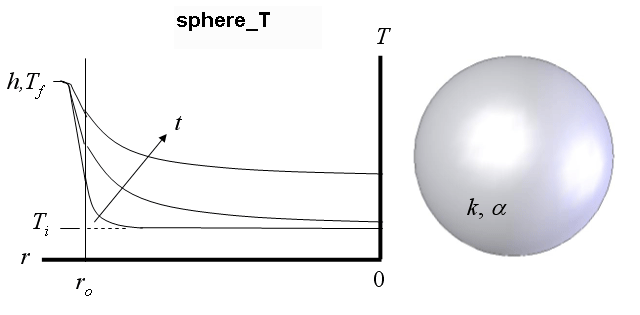Contents - Index

Sphere_TFunction sphere_T(r, time, T_i, T_f, alpha, k, h, r_o) returns the temperature ( [C], [K], [F], or [R]) within a sphere whose outer surface (r=r_o) is exposed to convection.

Inputs:
r - radial position [m] or [ft]
time - time relative to beginning of convection [sec]
T_i - initial temperature of sphere [C], [K], [F], or [R]
T_f - bulk fluid temperature [C], [K], [F], or [R]
alpha - thermal diffusivity [m^2/s] or [ft^2/hr]
k - thermal conductivity of sphere [W/m-K] or [Btu/hr-ft-R]
h - heat transfer coefficient [W/m^2-K] or [Btu/hr-ft^2-R]
r_o - outer radius of sphere [m] or [ft]

Notes:
Function sphere_T determines the dimensionless length, and non-dimensional Biot and Fourier numbers. It then calls the function sphere_T_ND, which determines theta as described in Section 3.5.2 of Nellis and Klein. Theta is then resolved by sphere into the temperature.

Example:
\$UnitSystem SI K Pa J
r=0.02 [m]
time=3000 [sec]
T_i=303 [K]
T_f=363 [K]
alpha=8.608e-5 [m^2/s]
k= 233 [W/m-K]
h= 45 [W/m^2-K]
r_o= 0.1 [m]
T=sphere_T(r, time, T_i, T_f, alpha, k, h, r_o)

{Solution: T=349.4 [K]}

Transient Conduction Index